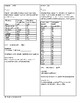# Circuit Training - Line of Best Fit (Linear Regression)Subject
Resource Type
File Type
PDF (1012 KB|4 pages)
Standards
\$2.50
• Product Description
• Standards

I wrote this for our high school algebra one students. Students will need technology (a graphing calculator with the "STAT" feature will be sufficient) to perform the 16 linear regressions. Students should be familiar with vocabulary such as correlation, relationship, causation, extrapolation, interpolation, linear, non-linear, prediction, correlation coefficient, though in all honesty, the circuit leads them through these ideas.

There is no answer key included since the answers are part of the circuit; it is how students advance from one question to the next. The only thing the teacher needs to do is work the circuit ahead of the students to decide how to best use it in the classroom. The students and the circuit will take care of the rest!

Teachers, should you get stuck or suspect there is an error, please email me at virginia.cornelius@gocommodores.org should you get stuck and I will help you.

Distinguish between correlation and causation.
Compute (using technology) and interpret the correlation coefficient of a linear fit.
Interpret the slope (rate of change) and the intercept (constant term) of a linear model in the context of the data.
Fit a linear function for a scatter plot that suggests a linear association.
Fit a function to the data; use functions fitted to data to solve problems in the context of the data.
Total Pages
4 pages